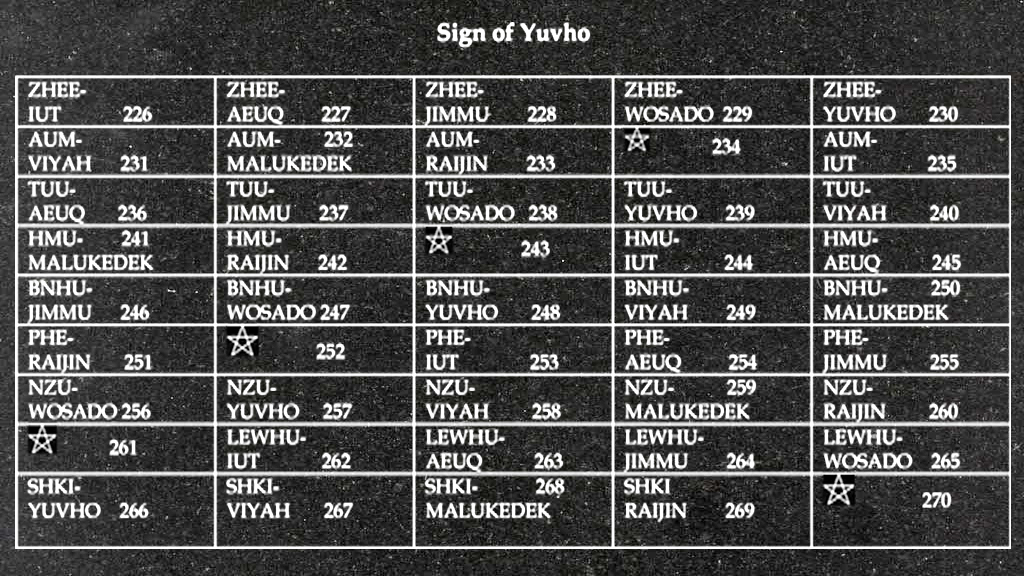The Sign of Yuvho -The Sign of Yuvho – Year 18,004 – Begins October 30th 2016

There are eight months in the Calendar of Mu, sometimes referred to as the Nyarzirian Calendar. Each month consists of forty-five days, and represents a system of the eight hexagrams moving through the five elements. The Ninzuwu Priest/Priestess invokes the mudra/mantra formulae associated with each hexagram that rules a particular day after invoking the Armor of Amaterasu Ohkami. The chart below illustrates the daily Vasuh letters invoked and the ruling “deity” for the said hexagram:
1. October 30th 2016 = Iut = 16th Hexagram = Aum-Hmu-Hmu
2. October 31st 2016 = Aeuq = 32nd Hexagram = Tuu-Aum
3. November 1st 2016 = Jimmu = 34th Hexagram = Tuu-Lewhu
4. November 2nd 2016 = Wosado = 40th Hexagram = Aum-Tuu-Tuu- Phe-Bnhu
5. November 3rd 2016 = Yuvho = 51st Hexagram = Zhee-Tuu-Aum-Shki
6. November 4th 2016 = Viyah = 54th Hexagram = Zhee-Lewhu-Aum-Zhee-Zhee
7. November 5th 2016 = Malukedek = 55th Hexagram = Aum-Lewhu
8. November 6th 2016 = Raijin = 62 Hexagram = Phe-Aum
9. November 7th 2016 == Entering Earth Element
10. November 8th 2016 = Iut = 16thHexagram = Aum-Hmu-Hmu
11. November 9th 2016 = Aeuq = 32ndHexagram = Tuu-Aum
12. November 10th2016 = Jimmu = 34thHexagram = Tuu-Lewhu
13. November 11th 2016 = Wosado = 40thHexagram = Aum-Tuu-Tuu- Phe-Bnhu
14. November 12th 2016 = Yuvho = 51stHexagram = Zhee-Tuu-Aum-Shki
15. November 13th 2016 = Viyah = 54thHexagram = Zhee-Lewhu-Aum-Zhee-Zhee
16. November 14th 2016 = Malukedek = 55thHexagram = Aum-Lewhu
17. November 15th 2016 = Raijin = 62 Hexagram = Phe-Aum
18. November 16th 2016 =Entering Water Element
19. November 17th 2016 = Iut = 16thHexagram = Aum-Hmu-Hmu
20. November 18th 2016 = Aeuq = 32ndHexagram = Tuu-Aum
21. November 19th 2016 = Jimmu = 34thHexagram = Tuu-Lewhu
22. November 20th  2016 = Wosado = 40thHexagram = Aum-Tuu-Tuu- Phe-Bnhu
23. November 21st 2016 = Yuvho = 51stHexagram = Zhee-Tuu-Aum-Shki
24. November 22nd  2016 = Viyah = 54thHexagram = Zhee-Lewhu-Aum-Zhee-Zhee
25. November 23rd 2016 = Malukedek = 55thHexagram = Aum-Lewhu
26. November 24th 2016 = Raijin = 62 Hexagram = Phe-Aum
27. November 25th 2016 =Entering Fire Element
28. November 26th 2016 = Iut = 16thHexagram = Aum-Hmu-Hmu
29. November 27th 2016 = Aeuq = 32ndHexagram = Tuu-Aum
30. November 28th 2016 = Jimmu = 34thHexagram = Tuu-Lewhu
31. November 29th 2016 = Wosado = 40thHexagram = Aum-Tuu-Tuu- Phe-Bnhu
32. November 30th 2016 = Yuvho = 51stHexagram = Zhee-Tuu-Aum-Shki
33. December 1st 2016 = Viyah = 54thHexagram = Zhee-Lewhu-Aum-Zhee-Zhee
34. December 2nd 2016 = Malukedek = 55thHexagram = Aum-Lewhu
35. December 3rd 2016 = Raijin = 62 Hexagram = Phe-Aum
36. December 4th  2016 =Entering Air Element
37. December 5th 2016 = Iut = 16thHexagram = Aum-Hmu-Hmu
38. December 6th 2016 = Aeuq = 32ndHexagram = Tuu-Aum
39. December 7th 2016 = Jimmu = 34thHexagram = Tuu-Lewhu
40. December 8th 2016 = Wosado = 40thHexagram = Aum-Tuu-Tuu- Phe-Bnhu
41. December 9th  2016 = Yuvho = 51stHexagram = Zhee-Tuu-Aum-Shki
42. December 10th 2016 = Viyah = 54thHexagram = Zhee-Lewhu-Aum-Zhee-Zhee
43. December 11th 2016 = Malukedek = 55thHexagram = Aum-Lewhu
44. December 12th 2016 = Raijin = 62 Hexagram = Phe-Aum
45. December 13th 2016 == Entering Void Element

## 3 thoughts on “Sign of Yuvho – Year 18,004: Begins October 30th 2016”

1.midnightsun888 says:

I am curious .I celebrate my Birthday of December 13, and I notice that we will be entering the void element. What does it mean?

2.midnightsun888 says: